# 手游特效太多怎么办？这里有一份性能优化方案可参考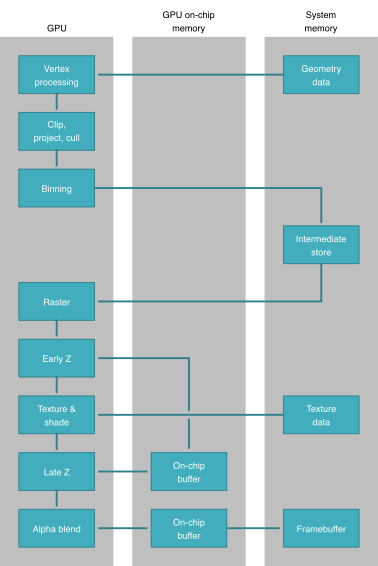HDR / MSAA

GPU 的 On-Chip Memory有非常高的读写速度，能大大提升MSAA/Alpha 混合的效率；但由于成本昂贵，因此 On-Chip Memory 的空间非常有限。例如从 Google 开源的 Andriod 驱动代码中可以得知，即使是旗舰级的 Adreno 630 亦只有 1 MiB 的 GMEM (即 Adreno 系列的 GPU On-Chip Memory)。[ 3 ]

· (1 + 1 + 1 + 1 + 2) Bytes 1 1920 * 1080 = 12441600 Bytes = 11.87MB

· 即需要拆分为 ~12个 Tile 来进行渲染

· (2 + 2 + 2 + 2 + 2) Bytes 4 1920 * 1080 = 82944000 Bytes = 79.10MB

· 即需要拆分为 ~80个 Tile 来进行渲染

Alpha 混合

UE4 的移动端渲染管线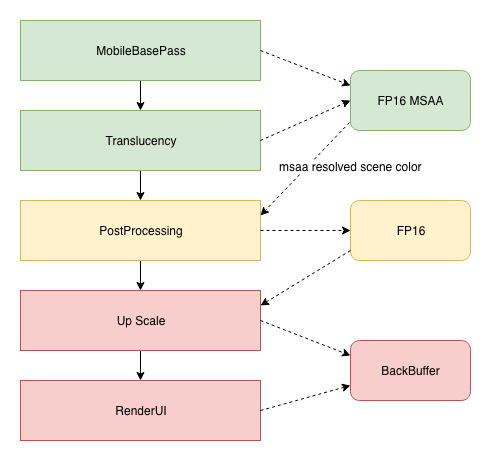MSAA 的特性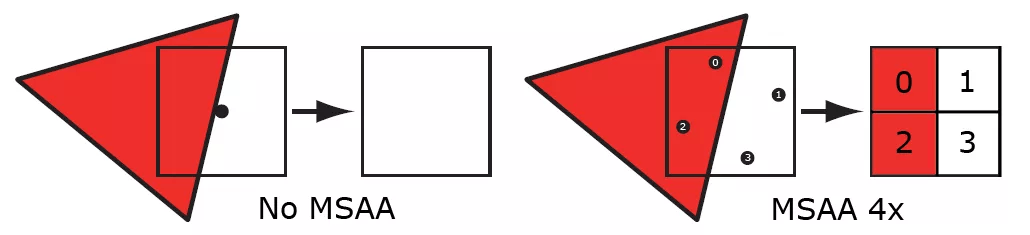[ 6 ]

d1 = d0 * (1 - a1) + s1 * a1;

d2 = d1 * (1 - a2) + s2 * a2;

d3 = d2 * (1 - a3) + s3 * a3;

d2 = [d0 * (1 - a1) * (1 - a2)] + [s1 * a1 * (1 - a2) + s2 * a2];

d3 = [d0 * (1 - a1) * (1 - a2) * (1 - a3)] + [s1 * a1 * (1 - a2) + s2 * a2] * (1 - a3)  + s3 * a3;

· [d0 * (1 - a1) * (1 - a2) * (1 - a3)]

· [s1 * a1 * (1 - a2) + s2 * a2] * (1 - a3) + s3 * a3

· Alpha 通道保存 fx.a = (1 - a1) * (1 - a2) * (1 - a3)

· RGB 通道则保存 fx.rgb = [s1 * a1 * (1 - a2) + s2 * a2] * (1 - a3) + s3 * a3

· 对应渲染特效的Blending Factors 则设为：

· AlphaBlendEnable = true;

· SrcBlend = SrcAlpha;

· DestBlend = InvSrcAlpha;

· SeparateAlphaBlendEnable = true;

· SrcBlendAlpha  = Zero;

· DestBlendAlpha = InvSrcAlpha;

·

· 由于在移动端 GPU 的浮点数 Alpha 混合比较慢 (在 S820 上以 1280 x 720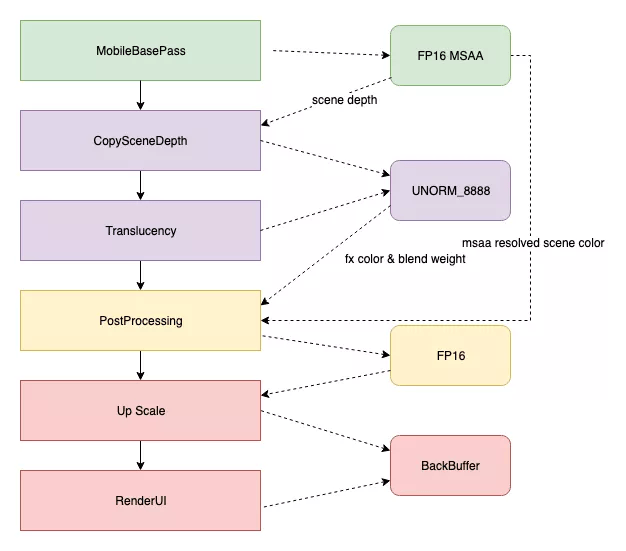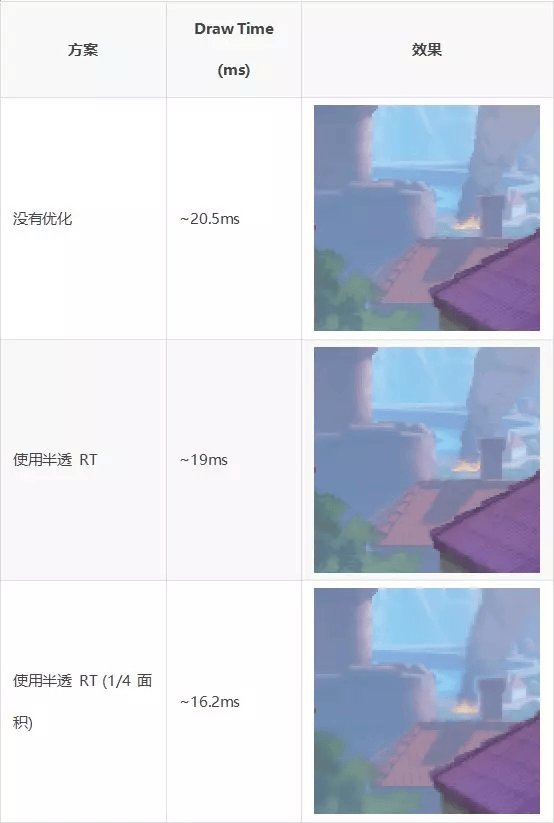·  三星：移动端 GPU Tiler 运作原理

·  三星：移动端 GPU 架构简介